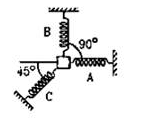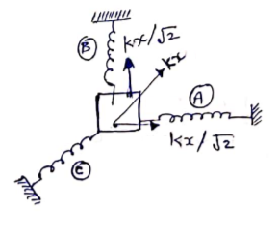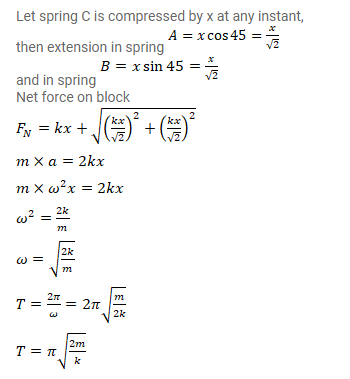# A particle of mass m is attached to three springs A,

Question:

A particle of mass $\mathrm{m}$ is attached to three springs $\mathrm{A}, \mathrm{B}$ and $\mathrm{C}$ of equal force constants $\mathrm{k}$ as shown in figure. If the particle is pushed slightly against the spring $C$ and released, find the time period of oscillation.Solution: